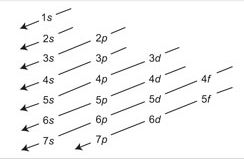# Describe on Aufbau Principle

Aufbau principle: Electrons first enter into lower energy orbital and subsequent electron enters into higher energy orbital gradually. Because lower energy orbital is more suitable than higher energy orbital.

According to Aufbau principle electrons first enter into lower energy orbital and subsequent electrons enter into higher energy orbital gradually. Because lower energy is more stable than higher energy orbital. In fact, the energy of an orbital is determined by the principal quantum numbers n and subsidiary quantum numbers l, with the help of an important rule (n + 1) rule or Bohr Bury’s rule.So for 4s orbital (n + l) = (4 + 0) = 4 Will be filled before 3d orbital (n + l) = (3 + 2) = 5 Consequently, last electron of K(19) enters 4s orbital instead of 3d orbital.

K(19) = 1s2 2s2 2p6 3s2 3p6 4s1